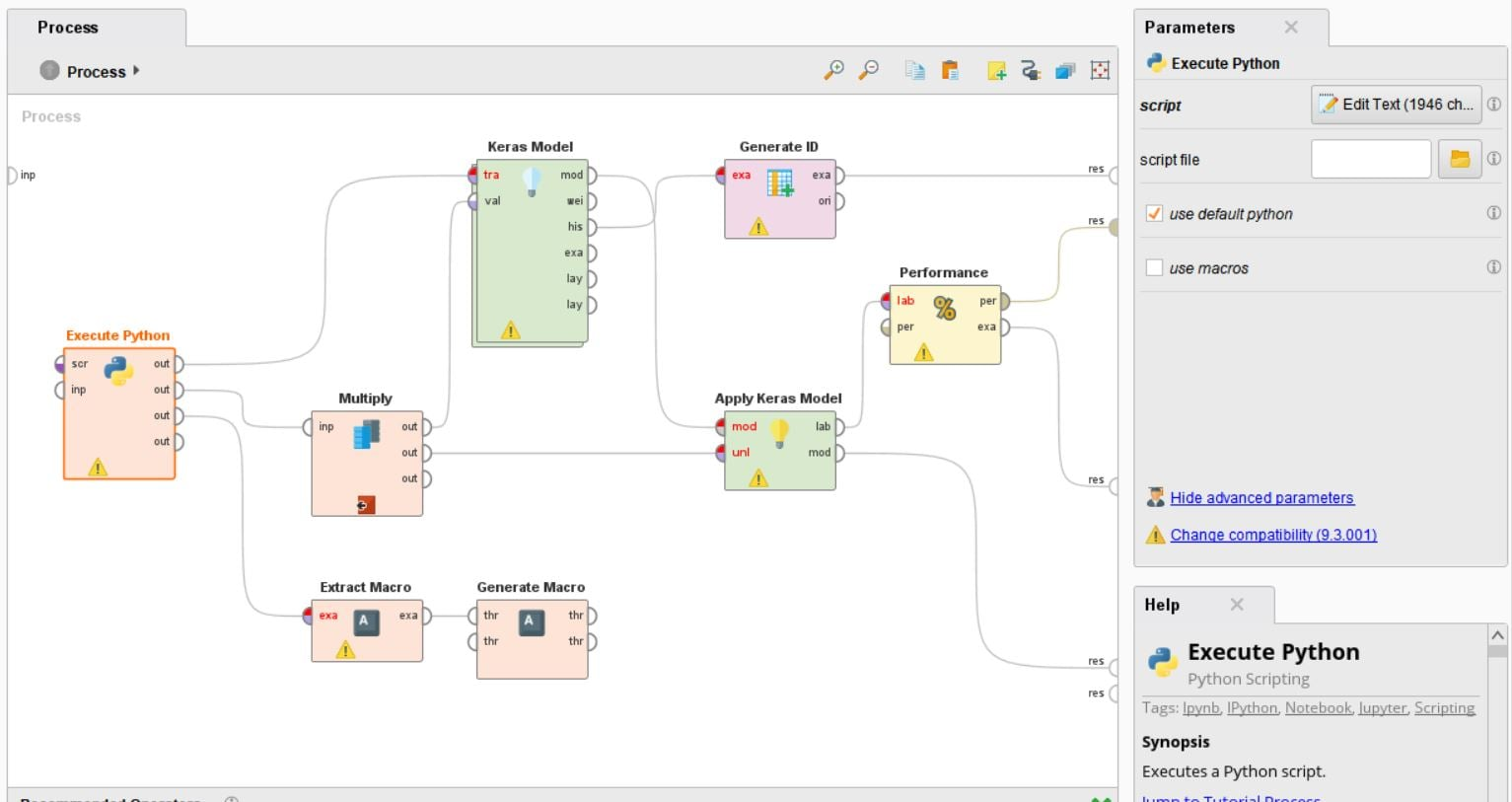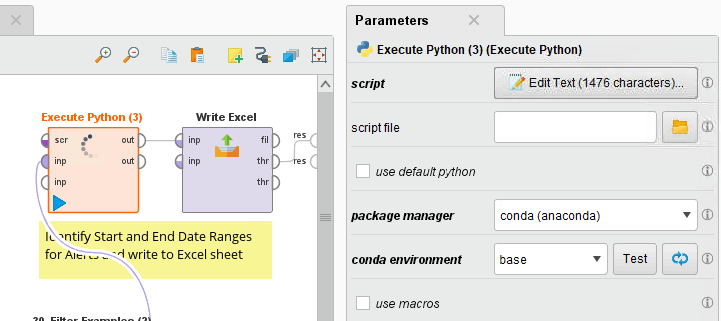# "Execute Python" operator takes forever to run

Member Posts: 9Newbie
in Help
I tried to implement the chapter 10 RapidMiner file from the classic RapidMiner textbook, 2nd edition by Vijay Kotu and Bala Deshpande. Dr Kotu is a member of the Advisory Board at RapidMiner.
(`http://www.introdatascience.com/download.html`)

Yes, I have installed the Execute Python operator from Marketplace. However, this operator takes forever to run. After waiting for more than 15 minutes, it still could not execute these codes. (However, I do not encounter this drag when using Jupyter).

Any idea if the Execute Python operator is not working?

The Python code is below, as copied from the textbook:

******************************************

from __future__ import print_function
import os
import numpy as np
import pandas as pd

import keras
from keras.datasets import mnist
from keras import backend as K

### Loads and returns MNIST data set in Pandas format
def rm_main():

# input image dimensions
img_rows, img_cols, ch_no = 28, 28, 1
num_classes = 10

# the data, shuffled and split between train and test sets
(x_train, y_train), (x_test, y_test) = mnist.load_data()

if K.image_data_format() == 'channels_first':
input_shape = (ch_no, img_rows, img_cols)
else:
input_shape = (img_rows, img_cols, ch_no)

x_train = x_train.reshape(x_train.shape, img_rows*img_cols*ch_no).astype('float32')
x_test = x_test.reshape(x_test.shape, img_rows*img_cols*ch_no).astype('float32')
x_train /= 255
x_test /= 255

# convert image vectors to data frames
df_train = pd.concat([pd.DataFrame(data={'y': y_train}), pd.DataFrame.from_dict(x_train)], axis=1)
df_test = pd.concat([pd.DataFrame(data={'y': y_test}), pd.DataFrame.from_dict(x_test)], axis=1)

# Prepare shape info
shape_data = np.array([['', 'rows', 'cols', 'ch', 'shape'],
['', img_rows, img_cols, ch_no, str(input_shape)]])

shape_result = pd.DataFrame(data=shape_data[1:,1:],
index=shape_data[1:,0],
columns=shape_data[0,1:])

# Return results
return df_train, df_test, shape_result

• Member Posts: 323Unicorn
edited May 2020
Does your process finish or does "take forever to run" mean that it does not finish at all? Have you tried the execute python operator without any additional code? It is much easier to post your process as these errors are quick to identify if we can see them first hand without having to read through code. As for the execution of the code itself, you would have to compare the execution time against the console. Python is being called in a separate thread so if you look in performance monitor, you will see the jvm and python working. There will be performance discrepancies  if you don't have enough memory or your cpu is saturated.
• Member Posts: 9Newbie
The Execute Python operator did not finish running even if I waited more than 15 minutes. For my sanity, I had to exit the software and relaunch again.

As requested, the XML is here. Hope you could spot the issues from this textbook example. Thank you.

==============================

<?xml version="1.0" encoding="UTF-8"?><process version="9.6.000">
<context>
<input/>
<output/>
<macros/>
</context>
<operator activated="true" class="process" compatibility="9.4.000" expanded="true" name="Process">
<parameter key="logverbosity" value="init"/>
<parameter key="random_seed" value="2001"/>
<parameter key="send_mail" value="never"/>
<parameter key="process_duration_for_mail" value="30"/>
<parameter key="encoding" value="SYSTEM"/>
<process expanded="true">
<operator activated="true" class="python_scripting:execute_python" compatibility="9.3.001" expanded="true" height="124" name="Execute Python" width="90" x="45" y="187">
<parameter key="notebook_cell_tag_filter" value=""/>
<parameter key="use_default_python" value="true"/>
<parameter key="package_manager" value="conda (anaconda)"/>
<parameter key="use_macros" value="false"/>
</operator>
<operator activated="true" class="extract_macro" compatibility="9.6.000" expanded="true" height="68" name="Extract Macro" width="90" x="246" y="391">
<parameter key="macro" value="img_shape"/>
<parameter key="macro_type" value="data_value"/>
<parameter key="statistics" value="average"/>
<parameter key="attribute_name" value="shape"/>
<parameter key="example_index" value="1"/>
<parameter key="img_rows" value="rows"/>
<parameter key="img_cols" value="cols"/>
<parameter key="img_channels" value="ch"/>
</list>
</operator>
<operator activated="true" class="generate_macro" compatibility="9.6.000" expanded="true" height="82" name="Generate Macro" width="90" x="380" y="391">
<list key="function_descriptions">
<parameter key="img_size" value="eval(%{img_rows})*eval(%{img_cols})*eval(%{img_channels})"/>
</list>
</operator>
<operator activated="true" breakpoints="before" class="multiply" compatibility="9.6.000" expanded="true" height="103" name="Multiply" width="90" x="246" y="238"/>
<operator activated="true" class="keras:sequential" compatibility="1.0.003" expanded="true" height="166" name="Keras Model" width="90" x="380" y="34">
<parameter key="input shape" value="(%{img_size},)"/>
<parameter key="loss" value="categorical_crossentropy"/>
<parameter key="learning rate" value="1.0"/>
<parameter key="momentum" value="0.0"/>
<parameter key="rho" value="0.95"/>
<parameter key="beta 1" value="0.999"/>
<parameter key="beta 2" value="0.999"/>
<parameter key="epsilon" value="1.0E-8"/>
<parameter key="decay" value="0.0"/>
<parameter key="schedule decay" value="0.004"/>
<parameter key="Nesterov" value="false"/>
<parameter key="use metric" value="true"/>
<enumeration key="metric">
<parameter key="metric" value="categorical_accuracy"/>
</enumeration>
<parameter key="epochs" value="12"/>
<parameter key="batch size" value="128"/>
<enumeration key="callbacks">
<parameter key="callbacks" value="TensorBoard(log_dir='/tmp/keras_logs/MNIST_RM', histogram_freq=0, write_graph=True, write_images=True, embeddings_freq=0, embeddings_layer_names=None, embeddings_metadata=None)"/>
</enumeration>
<parameter key="verbose" value="2"/>
<parameter key="validation split" value="0.0"/>
<parameter key="shuffle" value="true"/>
<parameter key="fix seed" value="false"/>
<parameter key="random seed" value="0"/>
<process expanded="true">
<operator activated="true" class="keras:core_layer" compatibility="1.0.003" expanded="true" height="82" name="Add Reshape" width="90" x="45" y="34">
<parameter key="layer_type" value="Reshape"/>
<parameter key="no_units" value="1"/>
<parameter key="activation_function" value="None"/>
<parameter key="use_bias" value="true"/>
<parameter key="kernel_initializer" value="glorot_uniform(seed=None)"/>
<parameter key="bias_initializer" value="Zeros()"/>
<parameter key="kernel_regularizer" value="None"/>
<parameter key="bias_regularizer" value="None"/>
<parameter key="activity_regularizer" value="None"/>
<parameter key="kernel_constraint" value="None"/>
<parameter key="bias_constraint" value="None"/>
<parameter key="rate" value="0.1"/>
<parameter key="noise_shape" value="None"/>
<parameter key="seed" value="None"/>
<parameter key="target_shape" value="%{img_shape}"/>
<parameter key="dims" value="1.1"/>
<parameter key="repetition_factor" value="1"/>
<parameter key="function" value="None"/>
<parameter key="l1" value="0.0"/>
<parameter key="l2" value="0.0"/>
</operator>
<operator activated="true" class="keras:conv_layer" compatibility="1.0.003" expanded="true" height="82" name="Add Conv2D 1" width="90" x="179" y="34">
<parameter key="layer_type" value="Conv2D"/>
<parameter key="filters" value="32"/>
<parameter key="kernel_size_1d" value="1"/>
<parameter key="kernel_size_2d" value="3.3"/>
<parameter key="kernel_size_3d" value="1.1.1"/>
<parameter key="strides_1d" value="1"/>
<parameter key="strides_2d" value="1.1"/>
<parameter key="strides_3d" value="1.1.1"/>
<parameter key="padding_2d" value="((1, 1), (1, 1))"/>
<parameter key="padding_3d" value="((1, 1), (1, 1), (1, 1))"/>
<parameter key="cropping_1d" value="1.1"/>
<parameter key="cropping_2d" value="((1, 1), (1, 1))"/>
<parameter key="cropping_3d" value="((1, 1), (1, 1), (1, 1))"/>
<parameter key="size_1d" value="2"/>
<parameter key="size_2d" value="2.2"/>
<parameter key="size_3d" value="2.2.2"/>
<parameter key="data_format" value="'channels_last'"/>
<parameter key="dilation_rate_1d" value="1"/>
<parameter key="dilation_rate_2d" value="1.1"/>
<parameter key="dilation_rate_3d" value="1.1.1"/>
<parameter key="depth_multiplier" value="1"/>
<parameter key="activation_function" value="'relu'"/>
<parameter key="use_bias" value="true"/>
<parameter key="kernel_initializer" value="glorot_uniform(seed=None)"/>
<parameter key="bias_initializer" value="Zeros()"/>
<parameter key="depthwise_initializer" value="glorot_uniform(seed=None)"/>
<parameter key="pointwise_initializer" value="glorot_uniform(seed=None)"/>
<parameter key="kernel_regularizer" value="None"/>
<parameter key="bias_regularizer" value="None"/>
<parameter key="activity_regularizer" value="None"/>
<parameter key="depthwise_regularizer" value="None"/>
<parameter key="pointwise_regularizer" value="None"/>
<parameter key="kernel_constraint" value="None"/>
<parameter key="bias_constraint" value="None"/>
<parameter key="depthwise_constraint" value="None"/>
<parameter key="pointwise_constraint" value="None"/>
</operator>
<operator activated="true" class="keras:conv_layer" compatibility="1.0.003" expanded="true" height="82" name="Add Conv2D 2" width="90" x="313" y="34">
<parameter key="layer_type" value="Conv2D"/>
<parameter key="filters" value="64"/>
<parameter key="kernel_size_1d" value="1"/>
<parameter key="kernel_size_2d" value="3.3"/>
<parameter key="kernel_size_3d" value="1.1.1"/>
<parameter key="strides_1d" value="1"/>
<parameter key="strides_2d" value="1.1"/>
<parameter key="strides_3d" value="1.1.1"/>
<parameter key="padding_2d" value="((1, 1), (1, 1))"/>
<parameter key="padding_3d" value="((1, 1), (1, 1), (1, 1))"/>
<parameter key="cropping_1d" value="1.1"/>
<parameter key="cropping_2d" value="((1, 1), (1, 1))"/>
<parameter key="cropping_3d" value="((1, 1), (1, 1), (1, 1))"/>
<parameter key="size_1d" value="2"/>
<parameter key="size_2d" value="2.2"/>
<parameter key="size_3d" value="2.2.2"/>
<parameter key="data_format" value="'channels_last'"/>
<parameter key="dilation_rate_1d" value="1"/>
<parameter key="dilation_rate_2d" value="1.1"/>
<parameter key="dilation_rate_3d" value="1.1.1"/>
<parameter key="depth_multiplier" value="1"/>
<parameter key="activation_function" value="'relu'"/>
<parameter key="use_bias" value="true"/>
<parameter key="kernel_initializer" value="glorot_uniform(seed=None)"/>
<parameter key="bias_initializer" value="Zeros()"/>
<parameter key="depthwise_initializer" value="glorot_uniform(seed=None)"/>
<parameter key="pointwise_initializer" value="glorot_uniform(seed=None)"/>
<parameter key="kernel_regularizer" value="None"/>
<parameter key="bias_regularizer" value="None"/>
<parameter key="activity_regularizer" value="None"/>
<parameter key="depthwise_regularizer" value="None"/>
<parameter key="pointwise_regularizer" value="None"/>
<parameter key="kernel_constraint" value="None"/>
<parameter key="bias_constraint" value="None"/>
<parameter key="depthwise_constraint" value="None"/>
<parameter key="pointwise_constraint" value="None"/>
</operator>
<operator activated="true" class="keras:pooling_layer" compatibility="1.0.003" expanded="true" height="82" name="Add Pooling Layer" width="90" x="447" y="34">
<parameter key="layer_type" value="MaxPooling2D"/>
<parameter key="pool_size_1d" value="2"/>
<parameter key="pool_size_2d" value="2.2"/>
<parameter key="pool_size_3d" value="2.2.2"/>
<parameter key="strides_1d" value="2"/>
<parameter key="strides_2d" value="2.2"/>
<parameter key="strides_3d" value="2.2.2"/>
<parameter key="data_format" value="None"/>
</operator>
<operator activated="true" class="keras:core_layer" compatibility="1.0.003" expanded="true" height="82" name="Add Dropout 1" width="90" x="581" y="34">
<parameter key="layer_type" value="Dropout"/>
<parameter key="no_units" value="1"/>
<parameter key="activation_function" value="None"/>
<parameter key="use_bias" value="true"/>
<parameter key="kernel_initializer" value="glorot_uniform(seed=None)"/>
<parameter key="bias_initializer" value="Zeros()"/>
<parameter key="kernel_regularizer" value="None"/>
<parameter key="bias_regularizer" value="None"/>
<parameter key="activity_regularizer" value="None"/>
<parameter key="kernel_constraint" value="None"/>
<parameter key="bias_constraint" value="None"/>
<parameter key="rate" value="0.25"/>
<parameter key="noise_shape" value="None"/>
<parameter key="seed" value="None"/>
<parameter key="target_shape" value=""/>
<parameter key="dims" value="1.1"/>
<parameter key="repetition_factor" value="1"/>
<parameter key="function" value="None"/>
<parameter key="l1" value="0.0"/>
<parameter key="l2" value="0.0"/>
</operator>
<operator activated="true" class="keras:core_layer" compatibility="1.0.003" expanded="true" height="82" name="Add Flatten" width="90" x="179" y="238">
<parameter key="layer_type" value="Flatten"/>
<parameter key="no_units" value="1"/>
<parameter key="activation_function" value="None"/>
<parameter key="use_bias" value="true"/>
<parameter key="kernel_initializer" value="glorot_uniform(seed=None)"/>
<parameter key="bias_initializer" value="Zeros()"/>
<parameter key="kernel_regularizer" value="None"/>
<parameter key="bias_regularizer" value="None"/>
<parameter key="activity_regularizer" value="None"/>
<parameter key="kernel_constraint" value="None"/>
<parameter key="bias_constraint" value="None"/>
<parameter key="rate" value="0.1"/>
<parameter key="noise_shape" value="None"/>
<parameter key="seed" value="None"/>
<parameter key="target_shape" value=""/>
<parameter key="dims" value="1.1"/>
<parameter key="repetition_factor" value="1"/>
<parameter key="function" value="None"/>
<parameter key="l1" value="0.0"/>
<parameter key="l2" value="0.0"/>
</operator>
<operator activated="true" class="keras:core_layer" compatibility="1.0.003" expanded="true" height="82" name="Add Dense 1" width="90" x="313" y="238">
<parameter key="layer_type" value="Dense"/>
<parameter key="no_units" value="128"/>
<parameter key="activation_function" value="'relu'"/>
<parameter key="use_bias" value="true"/>
<parameter key="kernel_initializer" value="glorot_uniform(seed=None)"/>
<parameter key="bias_initializer" value="Zeros()"/>
<parameter key="kernel_regularizer" value="None"/>
<parameter key="bias_regularizer" value="None"/>
<parameter key="activity_regularizer" value="None"/>
<parameter key="kernel_constraint" value="None"/>
<parameter key="bias_constraint" value="None"/>
<parameter key="rate" value="0.1"/>
<parameter key="noise_shape" value="None"/>
<parameter key="seed" value="None"/>
<parameter key="target_shape" value=""/>
<parameter key="dims" value="1.1"/>
<parameter key="repetition_factor" value="1"/>
<parameter key="function" value="None"/>
<parameter key="l1" value="0.0"/>
<parameter key="l2" value="0.0"/>
</operator>
<operator activated="true" class="keras:core_layer" compatibility="1.0.003" expanded="true" height="82" name="Add Dropout 2" width="90" x="447" y="238">
<parameter key="layer_type" value="Dropout"/>
<parameter key="no_units" value="1"/>
<parameter key="activation_function" value="None"/>
<parameter key="use_bias" value="true"/>
<parameter key="kernel_initializer" value="glorot_uniform(seed=None)"/>
<parameter key="bias_initializer" value="Zeros()"/>
<parameter key="kernel_regularizer" value="None"/>
<parameter key="bias_regularizer" value="None"/>
<parameter key="activity_regularizer" value="None"/>
<parameter key="kernel_constraint" value="None"/>
<parameter key="bias_constraint" value="None"/>
<parameter key="rate" value="0.5"/>
<parameter key="noise_shape" value="None"/>
<parameter key="seed" value="None"/>
<parameter key="target_shape" value=""/>
<parameter key="dims" value="1.1"/>
<parameter key="repetition_factor" value="1"/>
<parameter key="function" value="None"/>
<parameter key="l1" value="0.0"/>
<parameter key="l2" value="0.0"/>
</operator>
<operator activated="true" class="keras:core_layer" compatibility="1.0.003" expanded="true" height="82" name="Add Dense Softmax" width="90" x="581" y="238">
<parameter key="layer_type" value="Dense"/>
<parameter key="no_units" value="10"/>
<parameter key="activation_function" value="'softmax'"/>
<parameter key="use_bias" value="true"/>
<parameter key="kernel_initializer" value="glorot_uniform(seed=None)"/>
<parameter key="bias_initializer" value="Zeros()"/>
<parameter key="kernel_regularizer" value="None"/>
<parameter key="bias_regularizer" value="None"/>
<parameter key="activity_regularizer" value="None"/>
<parameter key="kernel_constraint" value="None"/>
<parameter key="bias_constraint" value="None"/>
<parameter key="rate" value="0.1"/>
<parameter key="noise_shape" value="None"/>
<parameter key="seed" value="None"/>
<parameter key="target_shape" value=""/>
<parameter key="dims" value="1.1"/>
<parameter key="repetition_factor" value="1"/>
<parameter key="function" value="None"/>
<parameter key="l1" value="0.0"/>
<parameter key="l2" value="0.0"/>
</operator>
<connect from_op="Add Dense Softmax" from_port="layers 1" to_port="layers 1"/>
<portSpacing port="sink_layers 1" spacing="0"/>
<portSpacing port="sink_layers 2" spacing="0"/>
</process>
</operator>
<operator activated="true" class="generate_id" compatibility="9.6.000" expanded="true" height="82" name="Generate ID" width="90" x="581" y="34">
<parameter key="create_nominal_ids" value="false"/>
<parameter key="offset" value="0"/>
</operator>
<operator activated="true" class="keras:apply" compatibility="1.0.003" expanded="true" height="82" name="Apply Keras Model" width="90" x="581" y="238">
<parameter key="batch_size" value="8"/>
<parameter key="verbose" value="1"/>
</operator>
<operator activated="true" class="performance_classification" compatibility="9.6.000" expanded="true" height="82" name="Performance" width="90" x="715" y="136">
<parameter key="main_criterion" value="first"/>
<parameter key="accuracy" value="true"/>
<parameter key="classification_error" value="false"/>
<parameter key="kappa" value="true"/>
<parameter key="weighted_mean_recall" value="false"/>
<parameter key="weighted_mean_precision" value="false"/>
<parameter key="spearman_rho" value="false"/>
<parameter key="kendall_tau" value="false"/>
<parameter key="absolute_error" value="false"/>
<parameter key="relative_error" value="false"/>
<parameter key="relative_error_lenient" value="false"/>
<parameter key="relative_error_strict" value="false"/>
<parameter key="normalized_absolute_error" value="false"/>
<parameter key="root_mean_squared_error" value="false"/>
<parameter key="root_relative_squared_error" value="false"/>
<parameter key="squared_error" value="false"/>
<parameter key="correlation" value="true"/>
<parameter key="squared_correlation" value="false"/>
<parameter key="cross-entropy" value="false"/>
<parameter key="margin" value="false"/>
<parameter key="soft_margin_loss" value="false"/>
<parameter key="logistic_loss" value="false"/>
<parameter key="skip_undefined_labels" value="true"/>
<parameter key="use_example_weights" value="true"/>
<list key="class_weights"/>
</operator>
<connect from_op="Execute Python" from_port="output 1" to_op="Keras Model" to_port="training set"/>
<connect from_op="Execute Python" from_port="output 2" to_op="Multiply" to_port="input"/>
<connect from_op="Execute Python" from_port="output 3" to_op="Extract Macro" to_port="example set"/>
<connect from_op="Extract Macro" from_port="example set" to_op="Generate Macro" to_port="through 1"/>
<connect from_op="Multiply" from_port="output 1" to_op="Keras Model" to_port="validation set"/>
<connect from_op="Multiply" from_port="output 2" to_op="Apply Keras Model" to_port="unlabelled data"/>
<connect from_op="Keras Model" from_port="model" to_op="Apply Keras Model" to_port="model"/>
<connect from_op="Keras Model" from_port="history" to_op="Generate ID" to_port="example set input"/>
<connect from_op="Generate ID" from_port="example set output" to_port="result 1"/>
<connect from_op="Apply Keras Model" from_port="labelled data" to_op="Performance" to_port="labelled data"/>
<connect from_op="Apply Keras Model" from_port="model" to_port="result 4"/>
<connect from_op="Performance" from_port="performance" to_port="result 2"/>
<connect from_op="Performance" from_port="example set" to_port="result 3"/>
<portSpacing port="source_input 1" spacing="0"/>
<portSpacing port="sink_result 1" spacing="0"/>
<portSpacing port="sink_result 2" spacing="21"/>
<portSpacing port="sink_result 3" spacing="189"/>
<portSpacing port="sink_result 4" spacing="126"/>
<portSpacing port="sink_result 5" spacing="0"/>
</process>
</operator>
</process>• Member Posts: 2Contributor I
Hello Team, could you please tell what was the solution provided in this case?

Our team is also facing similar issue where execute Python operator keeps on spinning but doesn't finish ; no errors.
Any help is appreciated.

Thanks,
Abhishek Bourai
• Administrator, Moderator, Employee, RapidMiner Certified Analyst, RapidMiner Certified Expert, University Professor Posts: 3,453RM Data Scientist
it is almost certain that your script just takes this long. Any chance you can add print statements into the script to write the progress to the log file?
Best,
Martin
- Sr. Director Data Solutions, Altair RapidMiner -
Dortmund, Germany
• Member Posts: 2Contributor I
Thanks for response @mschmitz. Apologies for the delay in response.
This block keeps spinning as shown below.
Since i am new to Rapidminer , could you please through a light on the - package manager and conda environment. Do i need to create a separate environment in Anaconda?Here is my Python script  - some Python and Numpy operations

import pandas as pd
import numpy as np

def rm_main(data):
data['Time_diff']=(data['Timestamp']-data['Timestamp'].shift())
data['Time_diff']=data['Time_diff'].dt.total_seconds()
data['Time_diff_binary'] = np.where(data['Time_diff']!=900, 1, 0)
data['CUMSUM'] = data['Time_diff_binary'].cumsum()

cols=['Start_Timestamp','End_Timestamp','Anomaly_Type']
#   new_df=pd.DataFrame(columns=['Start_Timestamp','End_Timestamp','Anomaly_Type','IS_1','IS_2','IS_3','IS_4'])
new_df=pd.DataFrame(columns=new_cols)

for idx, grp in data.groupby('CUMSUM'):
st_time = grp['Timestamp'].iloc
et_time = grp['Timestamp'].iloc[-1]
anomaly = grp['Anomaly'].iloc
selected_idx = grp['E_Distance'].idxmax()

dict_temp = {'Start_Timestamp' : st_time, 'End_Timestamp' : et_time, 'Anomaly_Type':anomaly}